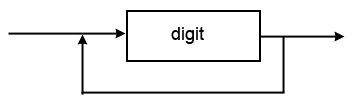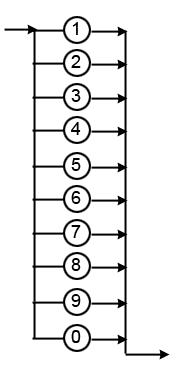# 6. Syntax Diagram example 1

#### Defining an integer using a syntax diagramThis indicates that an integer is made up of one or more digits. But note that digit itself needs further definition.

Defining a digit.Following the rules discussed earlier, you begin to read this diagram from the left. The arrow points to a number of parallel terminator symbols, but you can only follow a single path through them. Parallel symbols are effectively an 'OR' choice. In this case a digit is defined to consist of a single number ranging from zero to nine.

As a comparison, the BNF notation describes a digit as

digit :== 1 | 2 | 3 | 4 | 5 | 6 | 7 | 8 | 9 | 0

Therefore an integer can be defined using two syntax diagrams, one for the integer itself and another that describes a digit.

Challenge see if you can find out one extra fact on this topic that we haven't already told you

Click on this link: syntax diagrams